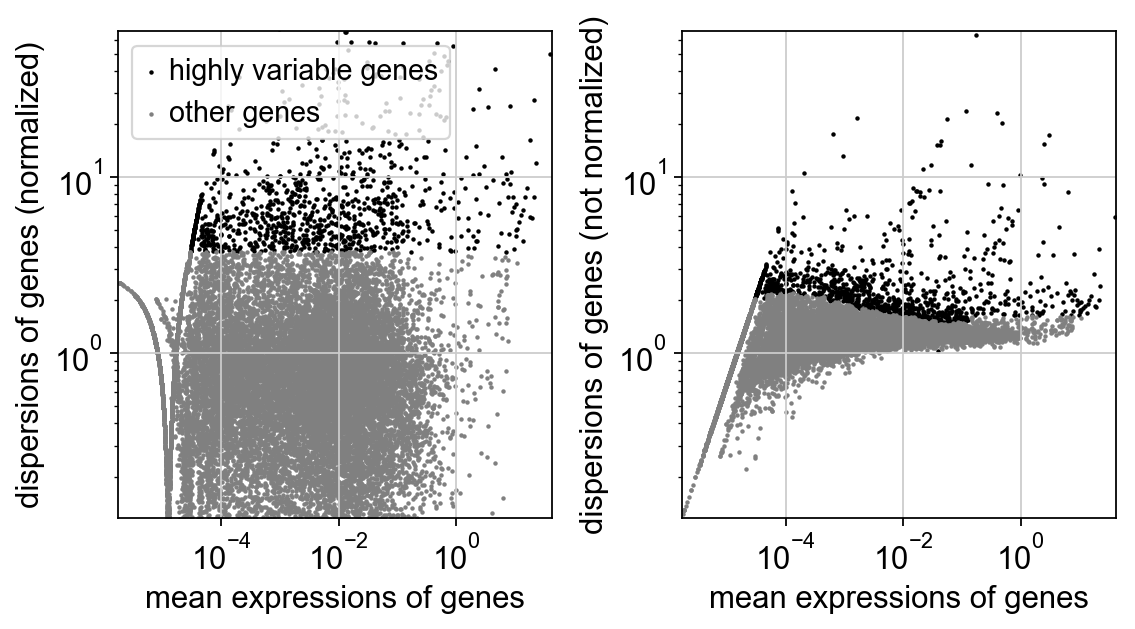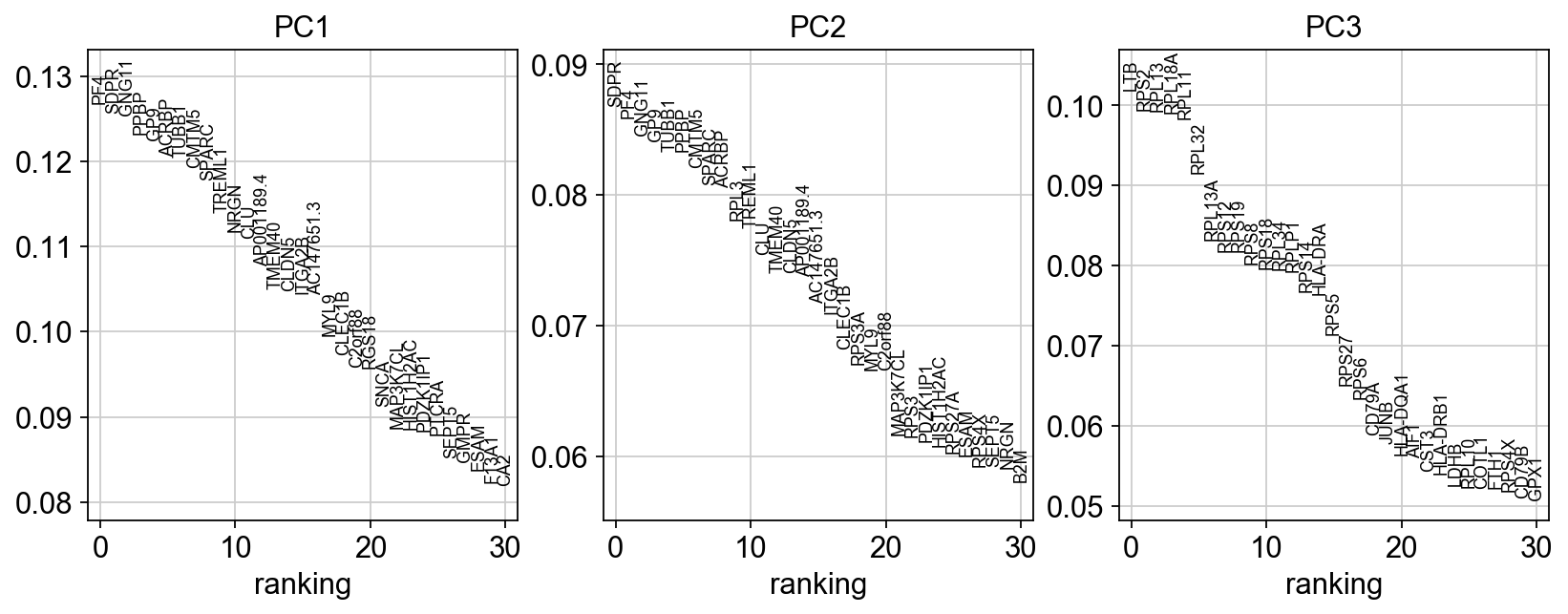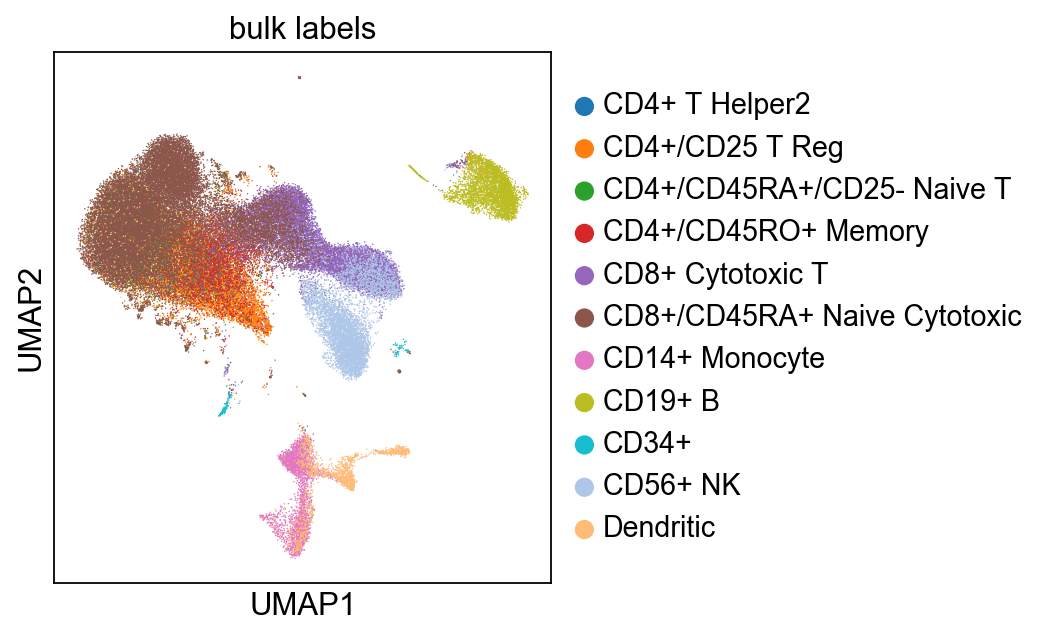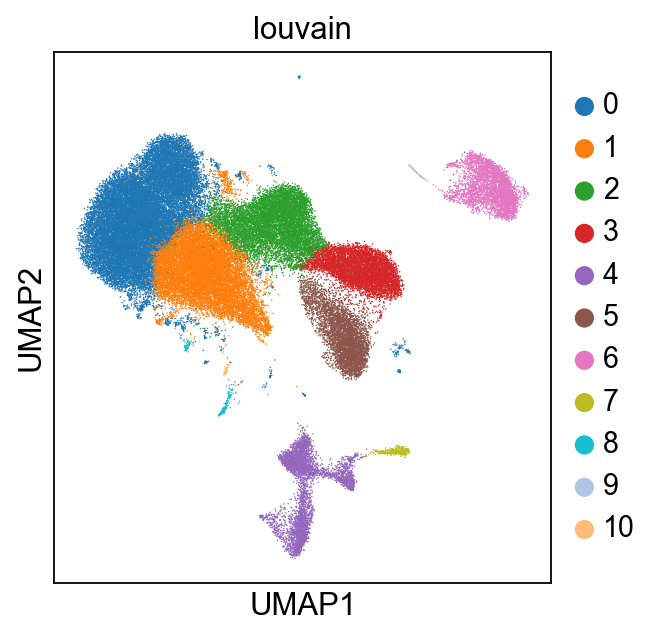First compiled: May 3, 2017. Updated Mar 28, 2018.
See the same notebook for Scanpy 0.2.7.

# Profiling Scanpy for 68k PBMC cells of Zheng et al., Nat. Comm. (2017)¶

This is the Scanpy benchmark for the Cell Ranger R analysis of Zheng et al., Nat. Comms. (2017) available from here. Compare the Scanpy version with the Cell Ranger version of this notebook.

The data is freely available [page/file] from the 10x homepage and from GitHub.

A more pedagogic tutorial can be found here.

In :
import numpy as np
import pandas as pd
import matplotlib.pyplot as pl
import scanpy.api as sc

sc.settings.verbosity = 1  # verbosity: errors (0), warnings (1), info (2), hints (3)
sc.settings.set_figure_params(dpi=80)  # low dpi (dots per inch) yields small inline figures
sc.logging.print_versions()

scanpy==1.0 anndata==0.5.8 numpy==1.14.1 scipy==1.0.0 pandas==0.22.0 scikit-learn==0.19.1 statsmodels==0.8.0 python-igraph==0.7.1 louvain==0.6.1


Initial usage of memory.

In :
sc.logging.print_memory_usage()

Memory usage: current 0.12 GB, difference +0.12 GB


Only use the first n cells, set to None if you want all cells.

In :
use_first_n_observations = None


Load the data. This takes a long time only when first reading the raw data from the .mtx text file. It's very fast through reading from the ./cache/ directory after that (change this using sc.settings.cachedir).

In :
%%time
path = './data/zheng17_filtered_matrices_mex/hg19/'

Variable names are not unique. To make them unique, call .var_names_make_unique.
CPU times: user 2.89 s, sys: 715 ms, total: 3.6 s
Wall time: 3.63 s

In :
adata.var_names_make_unique()


Let us use the cell type labels generated by Zheng et al. by correlating gene expression with purified bulk data. You can download the bulk labels here ./data/zheng17_bulk_lables.txt.

In :
adata.obs['bulk_labels'] = pd.read_csv('./data/zheng17_bulk_lables.txt', header=None).values


Reduce the number of observations for scaling information.

In :
adata = adata[:use_first_n_observations]


Save the logarithmized raw data for differential expression testing and plotting.

In :
sc.pp.log1p(adata, copy=True).write('./write/zheng17_raw.h5ad')

In :
adata

Out:
AnnData object with n_obs × n_vars = 68579 × 32738
obs: 'bulk_labels'

### Preprocessing¶

Instead of calling all steps of this preprocessing section separtely, you can call

sc.pp.recipe_zheng17(adata)

Per-cell normalize the data matrix $X$ and identify highly-variable genes.

In :
%%time
sc.pp.filter_genes(adata, min_counts=1)  # only consider genes with more than 1 count
sc.pp.normalize_per_cell(adata)          # normalize with total UMI count per cell
filter_result = sc.pp.filter_genes_dispersion(
sc.logging.print_memory_usage()

Memory usage: current 1.03 GB, difference +0.91 GB
CPU times: user 3.45 s, sys: 1.4 s, total: 4.85 s
Wall time: 5.02 s


Plot the dispersion relation. Use a logarithmic scale as the data is not yet logarithmized.

In :
sc.pl.filter_genes_dispersion(filter_result, log=True)Normalize the data again, logarithmize and scale the data. Then compute the PCA.

In :
%%time
sc.pp.normalize_per_cell(adata)  # need to redo normalization after filtering
sc.pp.log1p(adata)  # log transform: X = log(X + 1)
# the PCA is *not* contained in the recipe sc.pp.recipe_zheng17(adata)
sc.logging.print_memory_usage()

Memory usage: current 0.80 GB, difference -0.22 GB
CPU times: user 7.22 s, sys: 1.34 s, total: 8.56 s
Wall time: 6.05 s


Plot the PCA results.

In :
sc.pl.pca_loadings(adata)### Constructing and visualizing the single-cell graph¶

This will construct the single-cell graph - usually a knn graph - that describes cells in their relation to their neighbors.

In :
%%time
sc.logging.print_memory_usage()

Memory usage: current 0.90 GB, difference +0.10 GB
CPU times: user 27.9 s, sys: 3.05 s, total: 30.9 s
Wall time: 29.1 s

In :
%%time
sc.logging.print_memory_usage()

Memory usage: current 0.90 GB, difference +0.00 GB
CPU times: user 1min 15s, sys: 668 ms, total: 1min 15s
Wall time: 1min 11s

In :
sc.pl.umap(adata, color='bulk_labels')### Clustering the single-cell graph¶

Instead of providing a simple k-means clustering as in Cell Ranger, we use the Louvain algorithm of Blondel et al. (2008), as suggested by Levine et al. (2015) for single-cell analysis.

In :
%%time
sc.logging.print_memory_usage()

Memory usage: current 0.87 GB, difference -0.19 GB
CPU times: user 48.8 s, sys: 1.1 s, total: 49.9 s
Wall time: 50.7 s


Plot the clusters.

In :
sc.pl.umap(adata, color='louvain')### Marker Genes¶

Use the logarithmized raw data to rank genes according to differential expression.

In :
adata.raw = sc.read('./write/zheng17_raw.h5ad')

In :
%%time
sc.tl.rank_genes_groups(adata, 'louvain', groups=['1', '6', '9', '7'])
sc.logging.print_memory_usage()

Memory usage: current 1.44 GB, difference +0.57 GB
CPU times: user 6.95 s, sys: 3.71 s, total: 10.7 s
Wall time: 10.9 s

In :
sc.pl.rank_genes_groups(adata, n_genes=30)